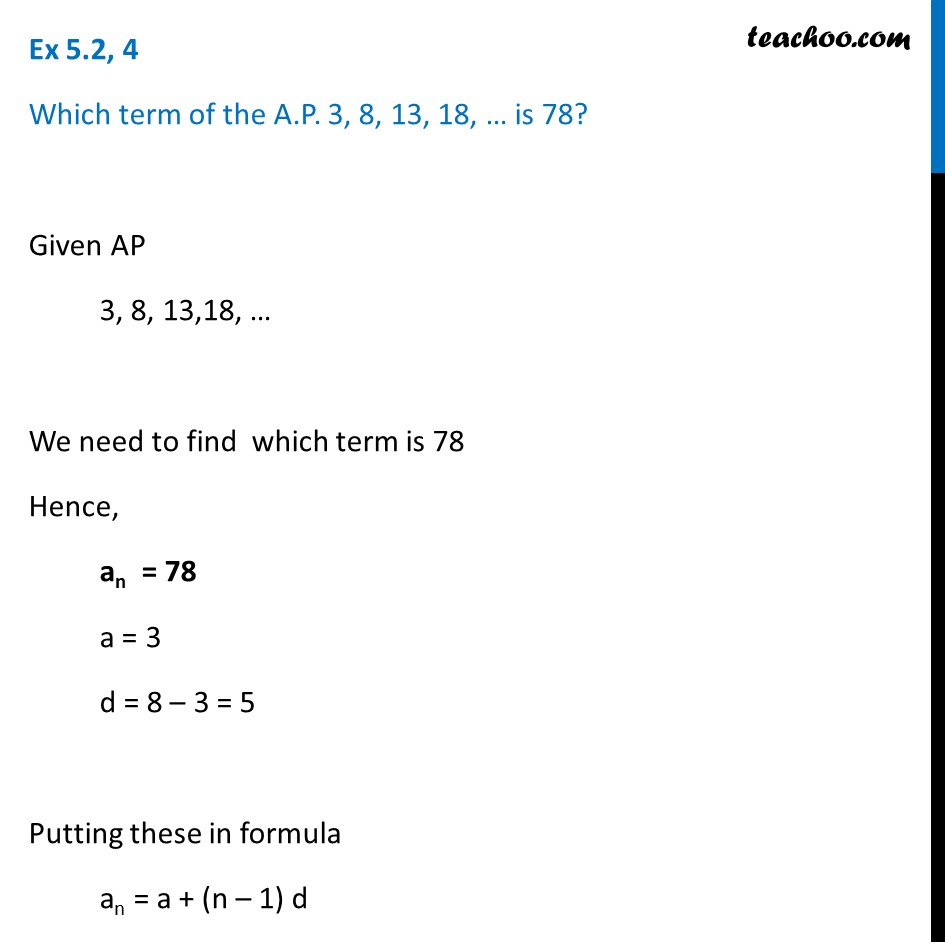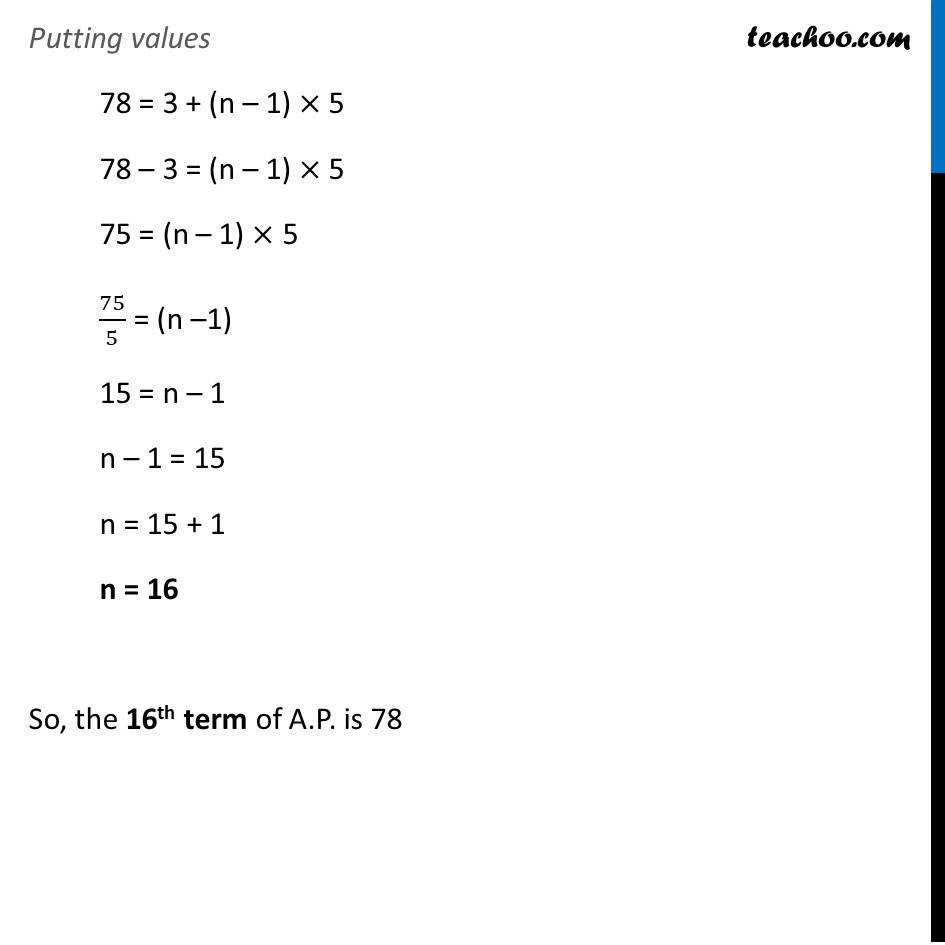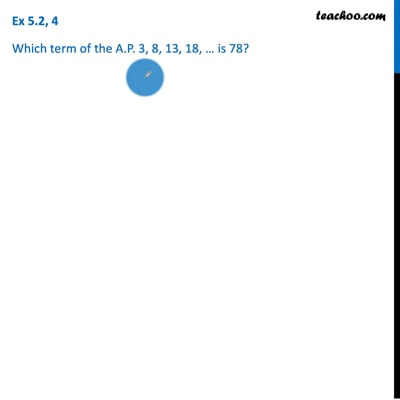Ex 5.2

Chapter 5 Class 10 Arithmetic Progressions
Serial order wiseThis video is only available for Teachoo black users

Learn in your speed, with individual attention - Teachoo Maths 1-on-1 Class

### Transcript

Ex 5.2, 4 Which term of the A.P. 3, 8, 13, 18, … is 78? Given AP 3, 8, 13,18, … We need to find which term is 78 Hence, an = 78 a = 3 d = 8 – 3 = 5 Putting these in formula an = a + (n – 1) d Putting values 78 = 3 + (n – 1) × 5 78 – 3 = (n – 1) × 5 75 = (n – 1) × 5 75/5 = (n –1) 15 = n – 1 n – 1 = 15 n = 15 + 1 n = 16 So, the 16th term of A.P. is 78